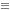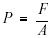Custom SearchThe equation is the most important concept in mathematics. Alone, algebraic operations are of little practical value. Only when these operations are coupled with algebraic equations can algebra be applied to solve practical problems.

An equation is a statement of equality between two equal quantities. Most people are familiar with the concept of equality. The idea of equal physical quantities is encountered routinely. An equation is merely the statement of this equality. There are three key ideas in an equation: an equation must involve two expressions, the expressions must be equal, and the equation must indicate that the expressions are equal. Thus, the statement that the sum of three and one equals four is an equation. It involves two expressions, (four and the sum of three and one), the expressions are equal, and the equation states that they are equal.

The equal sign (=) is used to indicate equality in an equation. In its most general form, an algebraic equation consists of two algebraic expressions separated by an equal sign. The equal sign is the key sign in algebra. It is the sign that defines one expression in terms of another. In solving practical problems, it is the sign that defines the unknown quantity in terms of known quantities.

Algebraic Equations

There are two kinds of equations: identities and conditional equations. An identity is an equation that is true for all values of the unknown involved. The identity sign () is used in place of the equal sign to indicate an identity. Thus, x2(x)(x), 3y + 5y8y, and yx + yz = y(x + z) are all identities because they are true for all values of x, y, or z. A conditional equation is one that is true only for some particular value(s) of the literal number(s) involved. A conditional equation is 3x + 5 = 8, because only the value x = 1 satisfies the equation. When the word equation is used by itself, it usually means a conditional equation.

The root(s) of an equation (conditional equation) is any value(s) of the literal number(s) in the equation that makes the equation true. Thus, 1 is the root of the equation 3x + 5 = 8 because x = 1 makes the equation true. To solve an algebraic equation means to find the root(s) of the equation.

The application of algebra is practical because many physical problems can be solved using algebraic equations. For example, pressure is defined as the force that is applied divided by the area over which it is applied. Using the literal numbers P (to represent the pressure), (to represent the force), and A (to represent the area over which the force is applied), this physical relationship can be written as the algebraic equation. When the numerical values of the force, F, and the area, A, are known at a particular time, the pressure, P, can be computed by solving this algebraic equation. Although this is a straightforward application of an algebraic equation to the solution of a physical problem, it illustrates the general approach that is used. Almost all physical problems are solved using this approach.Integrated Publishing, Inc. - A (SDVOSB) Service Disabled Veteran Owned Small Business